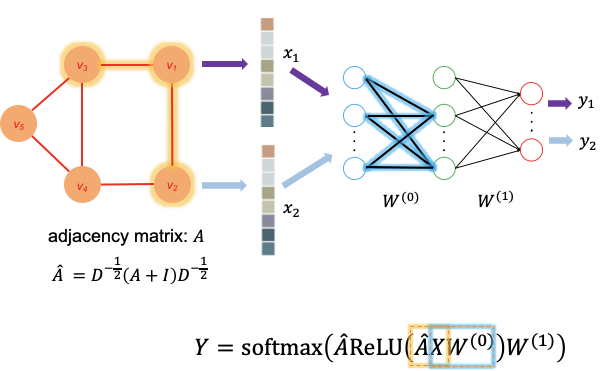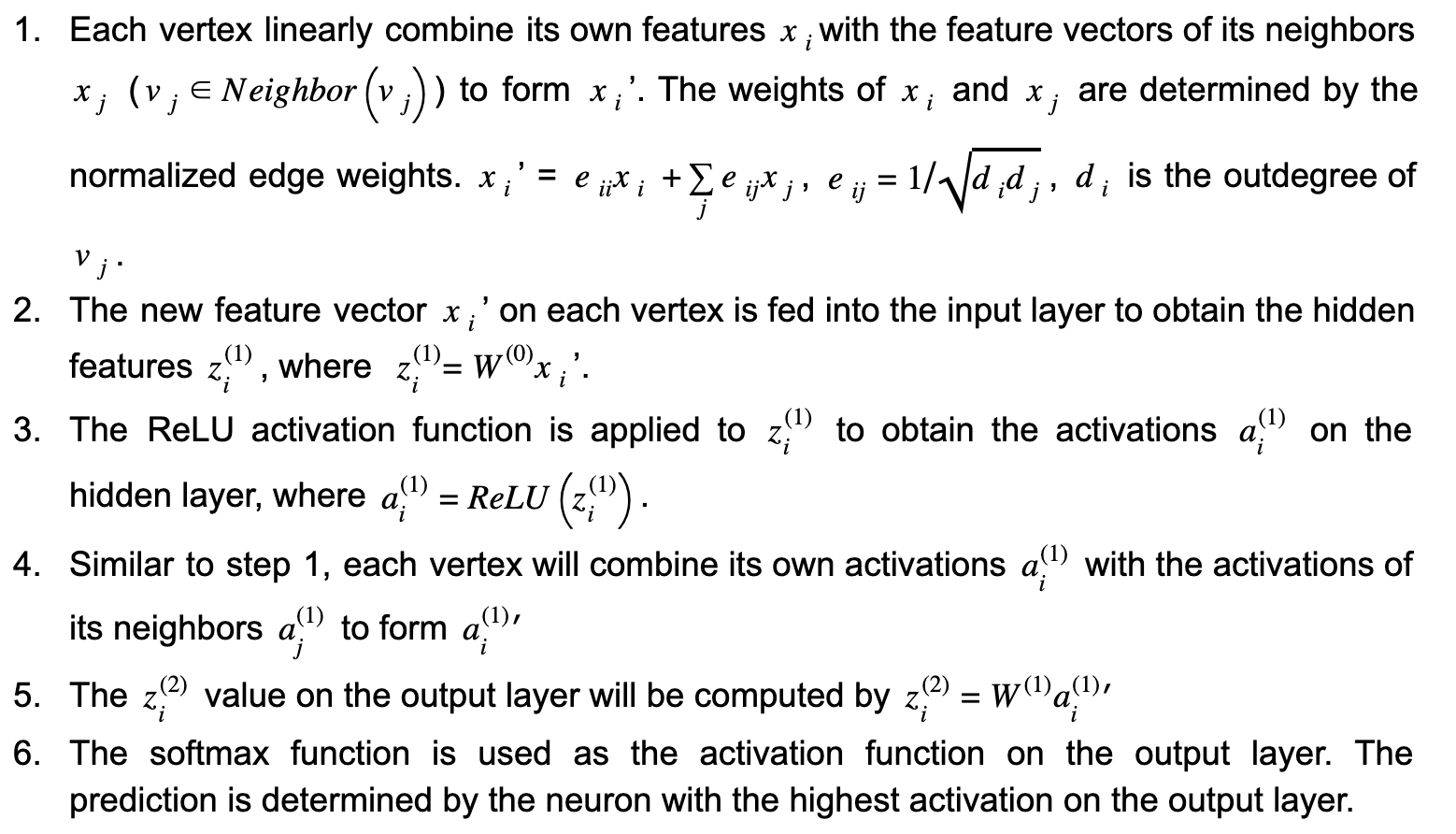# How to train graph convolutional network models in a graph databaseA typical feedforward neural network takes the features of each data point as input and outputs the prediction. The neural network is trained utilizing the features and the label of each data point in the training data set.

## What are graph convolutional networks?

A typical feedforward neural network takes the features of each data point as input and outputs the prediction. The neural network is trained utilizing the features and the label of each data point in the training data set. Such a framework has been shown to be very effective in a variety of applications, such as face identification, handwriting recognition, object detection, where no explicit relationships exist between data points. However, in some use cases, the prediction for a data point v(i) can be determined not only by its own features but also by the features of other data points v(j) when the relationship between v(i) and v(j) is given. For example, the topic of a journal paper (e.g computer science, physics, or biology) can be inferred from the frequency of words appearing in the paper. On the other hand, the reference in a paper can also be informative when predicting the topic of the paper. In this example, not only do we know the features of each individual data point (the word frequency), we also know the relationship between the data points (citation relation). So how can we combine them to increase the accuracy of the prediction?

By applying graph convolutional networks (GCN), the features of an individual data point and its connected data points will be combined and fed into the neural network. Let’s use the paper classification problem again as an example. In a citation graph (Fig. 1), each paper is represented by a vertex in the citation graph. The edges between the vertices represent the citation relationships. For simplicity, the edges are treated as undirected. Each paper and its feature vector are denoted as vi_ and xi_ respectively. Following the GCN model by Kipf and Welling , we can predict the topics of papers using a neural network with one hidden layer with the following steps:Figure 1.(Image by Author) The architecture of graph convolutional networks. Each vertex vi represents a paper in the citation graph. xi is the feature vector of vi. W(0) and W(1) are the weight matrices of the 3-layer neural network. A, D, and I are the adjacency matrix, outdegree matrix, and identity matrix respectively. The horizontal and vertical propagations are highlighted in orange and blue respectively.

In the above workflow, steps 1 and 4 perform horizontal propagation where the information of each vertex is propagated to its neighbors. While steps 2 and 5 perform vertical propagation where the information on each layer is propagated to the next layer. (see Fig. 1) For a GCN with multiple hidden layers, there will be multiple iterations of horizontal and vertical propagations. It is worth noting that each time horizontal propagation is performed, the information of a vertex is propagated one-hop further on the graph. In this example, the horizontal propagation is performed twice (steps 2 and 4), so the prediction of each vertex not only depends on its own features, but also the features of all the vertices within 2-hop distance from it. Additionally, since the weight matrix W(0) and W(1)are shared by all the vertices, the size of the neural network does not have to increase with the graph size, which makes this approach scalable.

## What is Supervised Machine Learning

What is neuron analysis of a machine? Learn machine learning by designing Robotics algorithm. Click here for best machine learning course models with AI

## Machine Learning | Everything you need to know

Machine learning is enabling computers to tackle tasks that have, until now, only been carried out by people. But what exactly is machine learning and what is making the current boom in machine learning possible?

## Pros and Cons of Machine Learning Language

AI, Machine learning, as its title defines, is involved as a process to make the machine operate a task automatically to know more join CETPA

## Graph Convolutional Networks on Node Classification

This article goes through the implementation of Graph Convolution Networks (GCN) using Spektral API, which is a Python library for graph deep learning based on Tensorflow 2.

## What is Machine learning and Why is it Important?

Machine learning is quite an exciting field to study and rightly so. It is all around us in this modern world. From Facebook’s feed to Google Maps for navigation, machine learning finds its application in almost every aspect of our lives. It is quite frightening and interesting to think of how our lives would have been without the use of machine learning. That is why it becomes quite important to understand what is machine learning, its applications and importance.